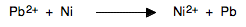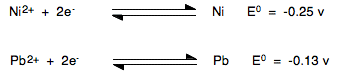Chemguide: Support for CIE A level Chemistry ``` ``` Learning outcome 24: Electrochemistry 24.2: Standard electrode potentials ``` ``` Learning outcome 24.2.10 This statement deals with the relationship between standard electrode potentials and Gibbs free energy. You won't be able to understand this unless you know about Gibbs free energy from statements in section 23.4. The statement is new to the syllabus for exams in 2022 onwards, and there isn't a question on it in the specimen papers. There is no way at the time of writing to judge what sort of questions CIE will ask. ``` ``` The key equation is: ΔG° = - nE°cellF You will often find this in books or on the web rearranged slightly as: ΔG° = - nFE°cell The terms: ΔG° is the standard Gibbs free energy change. n is the number of electrons transferred in the reaction. F is the Faraday constant, 96500 coulombs mol-1. E°cell is the standard cell potential in volts. ``` ``` We will look at a straightforward example of this, using what you should already know about calculating cell potentials from previous statements. Consider the reaction:If we split this into two half-reactions and find the standard electrode potentials for each of them, these are the E° values you would find from a data book:Note:  E° value equations are always written with the electrons on the left-hand side. Don't worry about the fact that in the overall equation we are looking at, we would need the nickel one the other way around. That sorts itself out in the equation E°cell = E°red - E°ox Here is the overall equation again:The Pb2+ ions are being reduced to Pb. The nickel is being oxidised to Ni2+ ions. Now we use the equation: E°cell = E°red - E°ox E°red is the value for the half-reaction where reduction is happening - that's the lead one. E°ox is for the oxidation half-reaction - that's the nickel one. E°cell = -0.13 - (-0.25) = +0.12 v You have a positive value for E°cell which you should remember is necessary for a reaction to happen. Now let's fit this into the equation this statement is talking about: ΔG° = - nE°cellF n is the number of electrons involved - in this case, 2. F is the Faraday constant, 96500 coulombs mol-1. ΔG° = - nE°cellF ΔG° = - 2 x 0.12 x 96500 = - 23160 J mol-1 ΔG° = - 23 kJ mol-1 to 2 sig figs Note:  The electrode potentials are only quoted to 2 significant figures, and so you can't quote your answer to more than that. You might well wonder where the unit joules comes from, and what happens to the unit v in the E°cell value. The answer is hidden in the coulombs. It turns out (this is physics - I had to look it up!) that a coulomb is a joule divided by a volt. That introduces the joule into the final units, and also gets rid of the volt unit from the electrode potentials. Now the really important bit Notice that the calculation has produced a negative value for ΔG°. That means that the reaction is feasible. You will only get that result if E°cell is positive. The minus sign in the equation ΔG° = - nE°cellF would turn a negative value for E°cell into a positive answer on that side of the equation. You would end up with a positive ΔG° value, and that would make the reaction not feasible. This equation links together the two conditions for feasibility you have met: E°cell has to be positive. ΔG° has to be negative. ``` ``` Go to the Section 24 Menu . . . To return to the list of learning outcomes in Section 24 Go to the CIE Main Menu . . . To return to the list of all the CIE sections Go to Chemguide Main Menu . . . This will take you to the main part of Chemguide. © Jim Clark 2020